Precalculus : Solve a System of Quadratic Equations

Example Questions

Example Question #1 : Solve A System Of Quadratic Equations

Which of the following could NOT be a possible number of solutions of a system of quadratic and linear equations?

0

3

How many times the graphs intersect

1

3

Explanation:

Recall that the solution of a system of equations is given by the intersection points of the graphs. Thus, this question is really asking how many times a parabola and a line can intersect. Visualize a parabola and a line. For this purpose, let's say that the parabola is facing up. If a line is drawn horizontally under the vertex, then it would not intersect the parabola at all, so the system would have no solutions. If the line is tangent to, or just skims the edge, of the parabola then it would only intersect once and the system would have one solution. If the line goes straight through the parabola, then it would intersect twice. There is no other option for the orientation of the line and parabola. Thus, there cannot be three solutions.

Example Question #2 : Solve A System Of Quadratic Equations

Find the coordinate of intersection, if possible: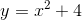and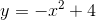.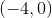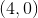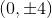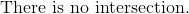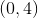Explanation:

To solve for x and y, set both equations equal to each other and solve for x.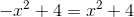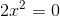Substituteinto either parabola.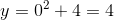The coordinate of intersection is.

Example Question #3 : Solve A System Of Quadratic Equations

Find the intersection(s) of the two parabolas: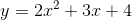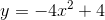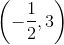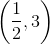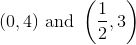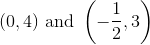Explanation:

Set both parabolas equal to each other and solve for x.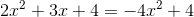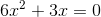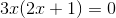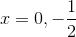Substitute both values ofinto either parabola and determine.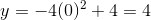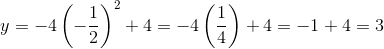The coordinates of intersection are:andExample Question #1 : Solve A System Of Quadratic Equations

Find the points of intersection: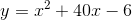;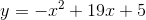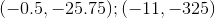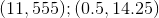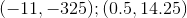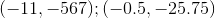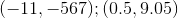Explanation:

To solve, set both equations equal to each other: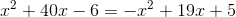To solve as a quadratic, combine like terms by adding/subtracting all three terms from the right side to the left side: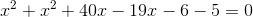This simplifies to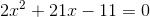Solving by factoring or the quadratic formula gives the solutions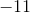and.

Plugging each into either original equation gives us: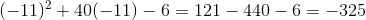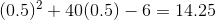Our coordinate pairs are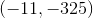and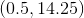.

Example Question #5 : Solve A System Of Quadratic Equations

Give the coordinate pairs that satisfy the system of equations.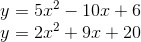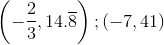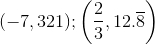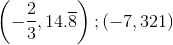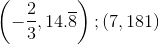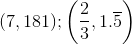Explanation:

To solve, set the two quadratics equal to each other and then combine like terms: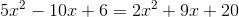subtract everything on the right from both sides to combine like terms.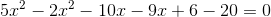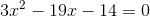Solving by factoring or using the quadratic formula gives us the solutions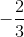and.

To find the y-coordinates, plug these into either equation: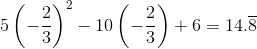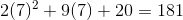Example Question #6 : Solve A System Of Quadratic Equations

Give the,coordinate pairs that satisfy the two equations.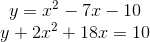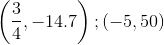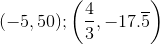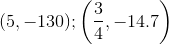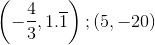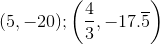Explanation:

To solve, first re-write the second one so that y is isolated on the left side: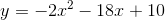Now set the two quadratics equal to each other: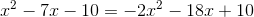add/subtract all of the terms from the right side so that this is a quadratic equal to zero.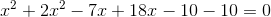combine like terms.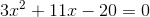Using the quadratic formula or by factoring, we get the two solutionsand.

To get the y-coordinates, plug these numbers into either function: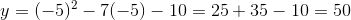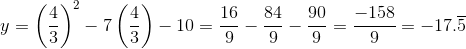Example Question #1 : Solve A System Of Quadratic Equations

Find the coordinate pairs satisfying both polynomials: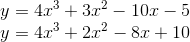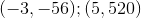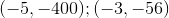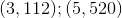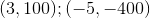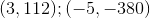Explanation:

To solve, set the two polynomials equal to each other: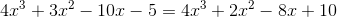add/subtract all of the terms from the right side from both sides.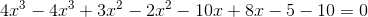combine like terms.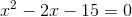Solving with the quadratic formula or by factoring gives us the solutions 5 and -3.

To get the y-coordinates, plug these numbers into either of the original equations: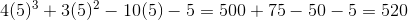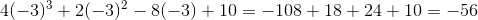All Precalculus Resources